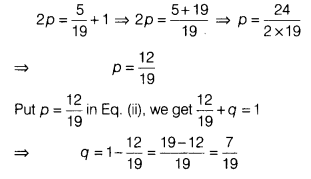# Find the probability of sucgess and failure of the event

If the difference between the probability of success and failure of an event is 5/19. Find the probability of sucgess and failure of the event.

Let P be the probability of success and q be the probability of failure.
Then, according to the question,
p-q = 5/19…(i)
Also, we know that, p + q = 1
On adding Eqs. (i) and (ii), we getHence, the probability of success is 12/19 and the probability of failure is 7/19